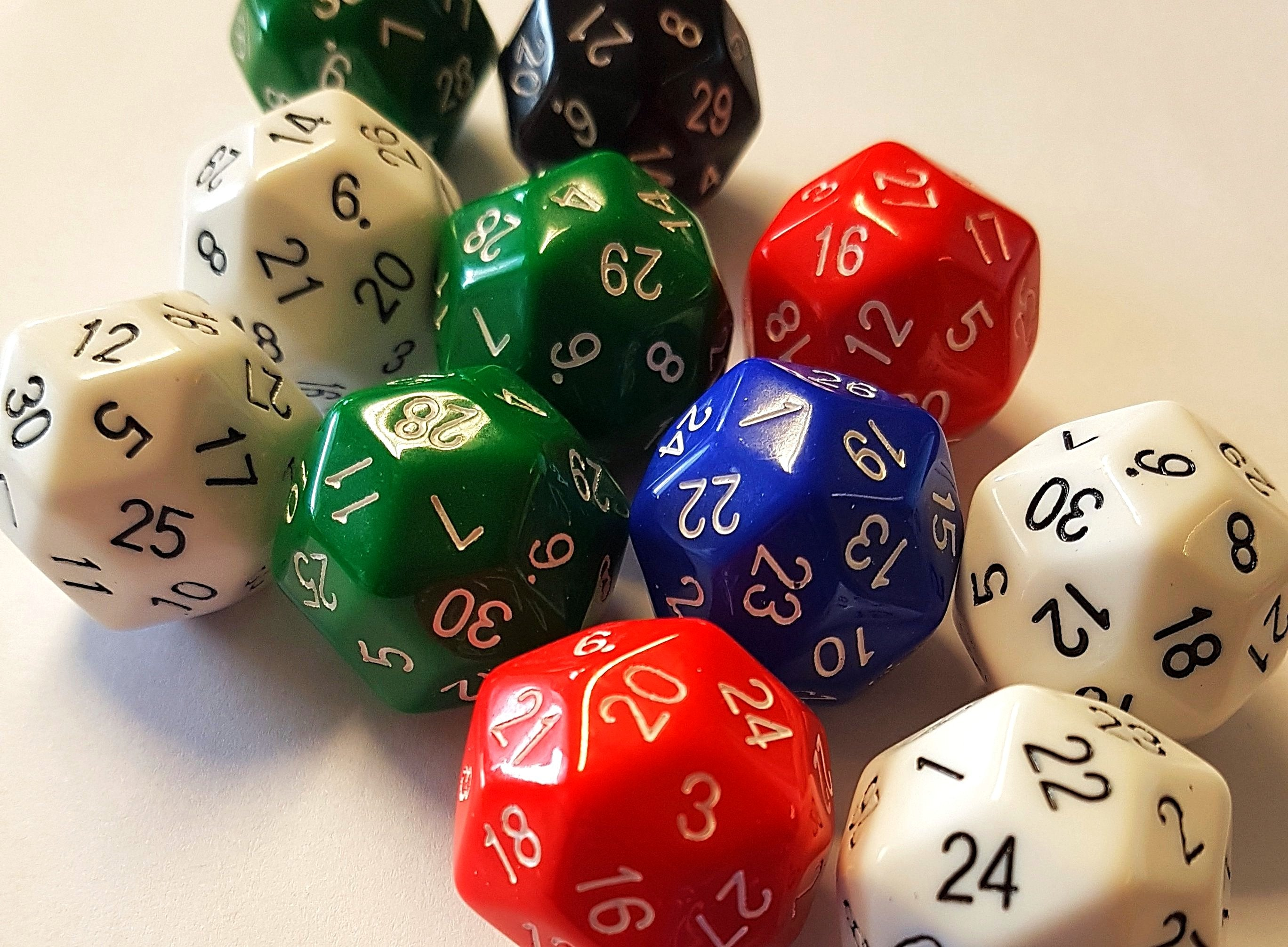1# Numerically Balanced D30

## £6.91

Behold the Numerically Balanced D30! The latest dice from The Dice Lab, makers of the Numerically Balanced D20.

This is a 30-sided die where not only do opposite faces always add to 31, but also numbers are evenly distributed around each vertex.

A D30 has 32 vertices of two types; 20 where three faces meet and 12 where five faces meet. On this ‘numerically balanced’ D30, ten of the three-face vertices have numbers around them summing to 46 and each of the other ten have a total of 47. Likewise the totals for each of the five-face vertices are split half and half between 77 and 78. This means there is no 'high side' or 'low side' to the dice, and makes the results rolled more evenly distributed.

If you want the numerically fairest D30 on the market: this is it.

Price = p125 pence = £6.91